GeeksforGeeks App
Open AppBrowser
Continue

# Merge two Pandas DataFrames on certain columns

We can merge two Pandas DataFrames on certain columns using the merge function by simply specifying the certain columns for merge.

Syntax: DataFrame.merge(right, how=’inner’, on=None, left_on=None, right_on=None, left_index=False, right_index=False, sort=False, copy=True, indicator=False, validate=None)

Example1: Let’s create a Dataframe and then merge them into a single dataframe.

Creating a Dataframe:

## Python3

 `# importing modules``import` `pandas as pd`` ` `# creating a dataframe``df1 ``=` `pd.DataFrame({``'Name'``:[``'Raju'``, ``'Rani'``, ``'Geeta'``, ``'Sita'``, ``'Sohit'``],``                    ``'Marks'``:[``80``, ``90``, ``75``, ``88``, ``59``]})`` ` `# creating another dataframe with different data``df2 ``=` `pd.DataFrame({``'Name'``:[``'Raju'``, ``'Divya'``, ``'Geeta'``, ``'Sita'``],``                    ``'Grade'``:[``'A'``, ``'A'``, ``'B'``, ``'A'``],``                    ``'Rank'``:[``3``, ``1``, ``4``, ``2` `],``                    ``'Gender'``:[``'Male'``, ``'Female'``, ``'Female'``, ``'Female'``]})``# display df1``display(df1)`` ` `# display df2``display(df2)`

Output: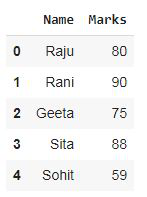df1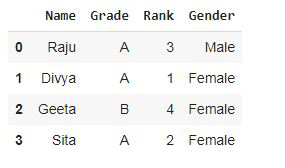df2

Now merge the dataframe:

## Python3

 `# applying merge``df1.merge(df2[[``'Name'``, ``'Grade'``, ``'Rank'``]])`

Output: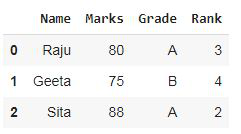Merged Dataframe

The resultant dataframe contains all the columns of df1 but certain specified columns of df2 with key column Name i.e. the resultant column contains Name, Marks, Grade, Rank column. Both dataframes has the different number of values but only common values in both the dataframes are displayed after merge.

Example 2: In the resultant dataframe Grade column of df2 is merged with df1 based on key column Name with merge type left i.e. all the values of left dataframe (df1) will be displayed.

## Python3

 `# importing modules``import` `pandas as pd`` ` `# creating a dataframe``df1 ``=` `pd.DataFrame({``'Name'``:[``'Raju'``, ``'Rani'``, ``'Geeta'``, ``'Sita'``, ``'Sohit'``],``                    ``'Marks'``:[``80``, ``90``, ``75``, ``88``, ``59``]})`` ` `# creating another dataframe with different data``df2 ``=` `pd.DataFrame({``'Name'``:[``'Raju'``, ``'Divya'``, ``'Geeta'``, ``'Sita'``],``                    ``'Grade'``:[``'A'``, ``'A'``, ``'B'``, ``'A'``],``                    ``'Rank'``:[``3``, ``1``, ``4``, ``2` `],``                    ``'Gender'``:[``'Male'``, ``'Female'``, ``'Female'``, ``'Female'``]})``# display df1``display(df1)`` ` `# display df2``display(df2)`` ` `# applying merge with more parameters``df1.merge(df2[[``'Grade'``, ``'Name'``]], on ``=` `'Name'``, how ``=` `'left'``)`

Output:df1df2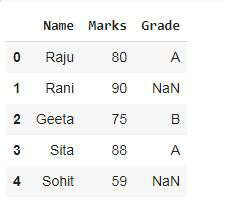Merged Dataframe

Example 3: In this example, we have merged df1 with df2. The Marks column of df1 is merged with df2 and only the common values based on key column Name in both the dataframes are displayed here.

## Python3

 `# importing modules``import` `pandas as pd`` ` `# creating a dataframe``df1 ``=` `pd.DataFrame({``'Name'``:[``'Raju'``, ``'Rani'``, ``'Geeta'``, ``'Sita'``, ``'Sohit'``],``                    ``'Marks'``:[``80``, ``90``, ``75``, ``88``, ``59``]})`` ` `# creating another dataframe with different data``df2 ``=` `pd.DataFrame({``'Name'``:[``'Raju'``, ``'Divya'``, ``'Geeta'``, ``'Sita'``],``                    ``'Grade'``:[``'A'``, ``'A'``, ``'B'``, ``'A'``],``                    ``'Rank'``:[``3``, ``1``, ``4``, ``2` `],``                    ``'Gender'``:[``'Male'``, ``'Female'``, ``'Female'``, ``'Female'``]})``# display df1``display(df1)`` ` `# display df2``display(df2)`` ` `# applying merge with more parameters``df2.merge(df1[[``'Marks'``, ``'Name'``]])`

Output:df1df2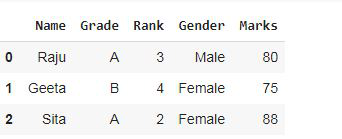Merged Dataframe

My Personal Notes arrow_drop_up